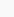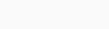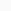# AP 9th Maths Mock Test 2021 For Chapter – 15 : “Proofs in Mathematics” Online Practice Test

1. "Every prime number is odd" . The counter example of this statement is .............
2. Match the following
1. All primes are odd                        ( ) a. 9
2. Every odd number is prime        ( ) b. 3 + 3 = 6
3. Sum of two odd numbers is odd( ) c. 2
3. "In any right angle the square of the smallest side equals to sum of the other sides" . Which of the following is a counter example of this statement ?
4. The product of three consecutive numbers is always divisible by................
5. If a , b are primes and N = a2b3 then the number of factors of N are
6. The product of two consecutive even number is always divisible by
7. "If 'n' is a whole number then 2n2 + 11 is prime" which of the following is a counter example for the statement ?
8. Which of the following types of sentences are not statements ?
9. 3x > x is a true statement if x satisfies
10. 3x + 1 > 4 becomes a true statement if x satisfies..............
11. Two times a real number x is even if ...............
12. Restate the following statements so that it becomes true ?
for any x , x30
13. A median is also an angle bisector in a ....................... triangle
14. Assertion A ; If x > y then x2 > y2 is not always true
Reason R : -2 > -3 and (-2)2 < (-3)2
15. Which of the following are true ?
16. Human beings are mortal . Jeevan is a human being . Based on these two statements , what can you say using deductive reasoning ?
17. Martians have red tongues . Gulag is a Martian . Based on these statements , what can you conclude ?
18. Given a polynomial P(x) = x2 + x + 41 then for which values of x , P(x) is a prime number ?
19. The statement "Every composite number can be expressed as a product of primes" is ......................
20. We can prove that the statement "sum of the interior angles of a triangle is 1800" by ..............
21. Mathematical statements are
22. Which of the following sentences are statements ?
a. x < y                     b. 3 + 2 = 5                      c. 4 > 8                         d. How are you ?
23. Which of the following is not a mathematical statement ?
24. An example which proves a given statement is false called
25. A statement or idea which gives an explanation to a series of observations is called....................
26. The main logical tool used in establishing the truth of an unambiguous statement is ...........
27. A mathematical statement whose truth has been established is called a ................
28. A statement which we believe is true , based on our mathematical intuition is called
29. Very helpful reasoning technique for making conjecture is .................
30. A conjecture may turn out to be ........
31. "Every even number greater than 4 can be written as sum of two prime numbers" this is called ................ 's conjecture
32. Drawing a conclusion based on patterns is called ................ reasoning
33.
1. Axiom         ( ) a. There passes infinitely many lines through a given point
2. Theorem     ( ) b. For every real x , P(x) = x2 + x + 41 is prime
3. Conjecture  ( ) c. The sum of interior angles of a triangle is 1800
34.
1. Forming conclusion based on patterns                          ( ) a. Disproof
2. Giving counter example to a statement                         ( ) b. Conjecture
3. Which we believe to be true                                             ( ) c. Inductive reasoning
35. Which of the following is a mathematical statement ?
36. You have given four cards . Each card has a number printed on one side and a letter on other side . "If a card has a consonant on one side , then it has an odd number on the other side" . Then which are the only two cardss you need to turn over to check whether the given rule holds ?37. What can you say about line - 5 ?
38. What can you say about line - 6 ?
39. For which lines of the above your conjectures hold good ?
40.
1. 28 = 2271 thus number of factors of 28 = (2 + 1)(1 + 1) = 32 = 6
2. 30 = 213151 thus number of factors of 30 = (1 + 1)(1 + 1)(1 + 1) = 222 = 8
From the above data , Number of factors of 24 is
41.
1. 28 = 2271 thus number of factors of 28 = (2 + 1)(1 + 1) = 32 = 6
2. 30 = 213151 thus number of factors of 30 = (1 + 1)(1 + 1)(1 + 1) = 222 = 8
From the above data , How many number of factors are there for 36 ?
42.
1. 28 = 2271 thus number of factors of 28 = (2 + 1)(1 + 1) = 32 = 6
2. 30 = 213151 thus number of factors of 30 = (1 + 1)(1 + 1)(1 + 1) = 222 = 8
From the above data , If N = ap.bq.cr where a , b , c are primes then number of factors of N = .............
43. If 12 = 1 , 112 = 121 , 1112 = 12321 then guess 111112
44. There is a 1010 table in which 0 to 9 are filled in the boxes of first row and 10 to 19 in the boxes of second row and so on in the last row 90 to 99 are filled in order.Here 8 clues are given to find a number in the puzzle
1. The number is greater than 9
2. The number is not a multiple 10
3. It is a multiple of 7
4. It is odd
5. It is not a multiple of 11
6. It is less than 200
7. Its ones digit is larger than its tens digit
8. Its tens digit is odd
Now what is the number ?
45. There is a 1010 table in which 0 to 9 are filled in the boxes of first row and 10 to 19 in the boxes of second row and so on in the last row 90 to 99 are filled in order.Here 8 clues are given to find a number in the puzzle
1. The number is greater than 9
2. The number is not a multiple 10
3. It is a multiple of 7
4. It is odd
5. It is not a multiple of 11
6. It is less than 200
7. Its ones digit is larger than its tens digit
8. Its tens digit is odd
Now which clues are useful ?
46. There is a 1010 table in which 0 to 9 are filled in the boxes of first row and 10 to 19 in the boxes of second row and so on in the last row 90 to 99 are filled in order.Here 8 clues are given to find a number in the puzzle
1. The number is greater than 9
2. The number is not a multiple 10
3. It is a multiple of 7
4. It is odd
5. It is not a multiple of 11
6. It is less than 200
7. Its ones digit is larger than its tens digit
8. Its tens digit is odd
Now which clues are not useful ?
47. The product of two even numbers is an ............. number
48. The sum of two odd numbers is
49. For each counting number x ,
50. All ....... angles are equal to one another
51. Straight lines parallel to the same straight line are .............. to one other
52. He is wise is
53. There are 27 days in month , it is a
54. Makarasankranthi falls on a Friday , it is a
55. The temperature in Hyderabad is 20 C , it is a
56. The earth is the only planet where life exist , it is a
57. Dog can fly , it is a
58. February has only 28 days , it is a
59. The sum of the interior angles of a quadrilateral is 3500
60. For any real number x , x20
61. A rhombus is a parallelogram
62. The sum of two even numbers is even
63. Square numbers can be written as the sum of two odd numbers
64. Restate the following
All numbers can be represented as the product of prime factors
65. Two times a real number is always
66. Restart the following statement
For any x , 3x + 1 > 4
67. In every ...................... triangle , a median is also an angle bisector
68. A mathematical statement whose truth has been established or proved is called
69. Based on our mathematical intuition , we believe a statement is true , but actually we are yet to prove it . Such statement is called
70. The sentences that can be judged on same criteria , no matter by what process for their being true or false are called
71. Which of the following are Axioms ?
72. A conjecture may be proved
73. The prime logical method in proving a mathematical statement is
74. Today is not Sunday , this is a
75. If 12 = 1 , 112 = 121 , 1112 = 12321 then guess 111112 =
76. Forming conclusion based on patterns is a
77. Giving counter example to a statement
78. Which we believe to be true is
79. Hypothesis means
80. Deductive reasoning means
81. Theorem means
82. Inductive means
83. Which of the following are related to Inductive ?
84. There passes infinitely many lines through a given point is
85. The sum of interior angles of a triangle is 1800 is
86. Which of the following are not a mathematical statement ?
87. Human beings are mortal . Jeevitha is human being , Based on these two statements , what can you say using deductive reasoning ?
88. Mathematics is based on
89. Science is based on
90. Generally Deductive reasoning has ............. types
91. A process which can establish the ytruth of mathematical statement based on purely on logical arguments is called
92. In axioms halves of equal things are
93. 2 is even number as
94. "Every prime number is odd" which of the following is not a counter example of this statement ?
95. Which of the following is odd according to in right angle the square of the smallest side equals to sum of other sides ?
96. The product of three consecutive numbers is always divisible by
97. The product of two consecutive even numbers is always divisible by
98. Which of the following types are statements ?
99. All Telugu people are Indians . X is an Indian . Can we conclude that X belongs to Telugu People
100. Beginning with hypothesis of the theorem and arrive at the conclusion by means of a chain of logical steps is mostly followed to prove